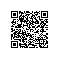# OpenCV中读取视频帧像素值的一般方法

OpenCV中读取视频帧像素值的一般方法可以分为以下几个步骤：

1、打开AVI格式的视频

CvCapture *capture=NULL;
IplImage *img=NULL;

if(!capture)
{
return;
}

2、从摄像头或者文件中顺序抓取并返回一帧

img=cvQueryFrame( capture );

3、读取当前帧像素值的基本方法

for(y = 0; y < height; y++)
{
for(x=0;x < width; x++)
{
UInt pixelB=(UInt)((uchar*)(img->imageData+img->widthStep*y))[3*x];
UInt pixelG=(UInt)((uchar*)(img->imageData+img->widthStep*y))[3*x+1];
UInt pixelR=(UInt)((uchar*)(img->imageData+img->widthStep*y))[3*x+2];
}
}使用钉钉扫一扫加入圈子
+ 订阅